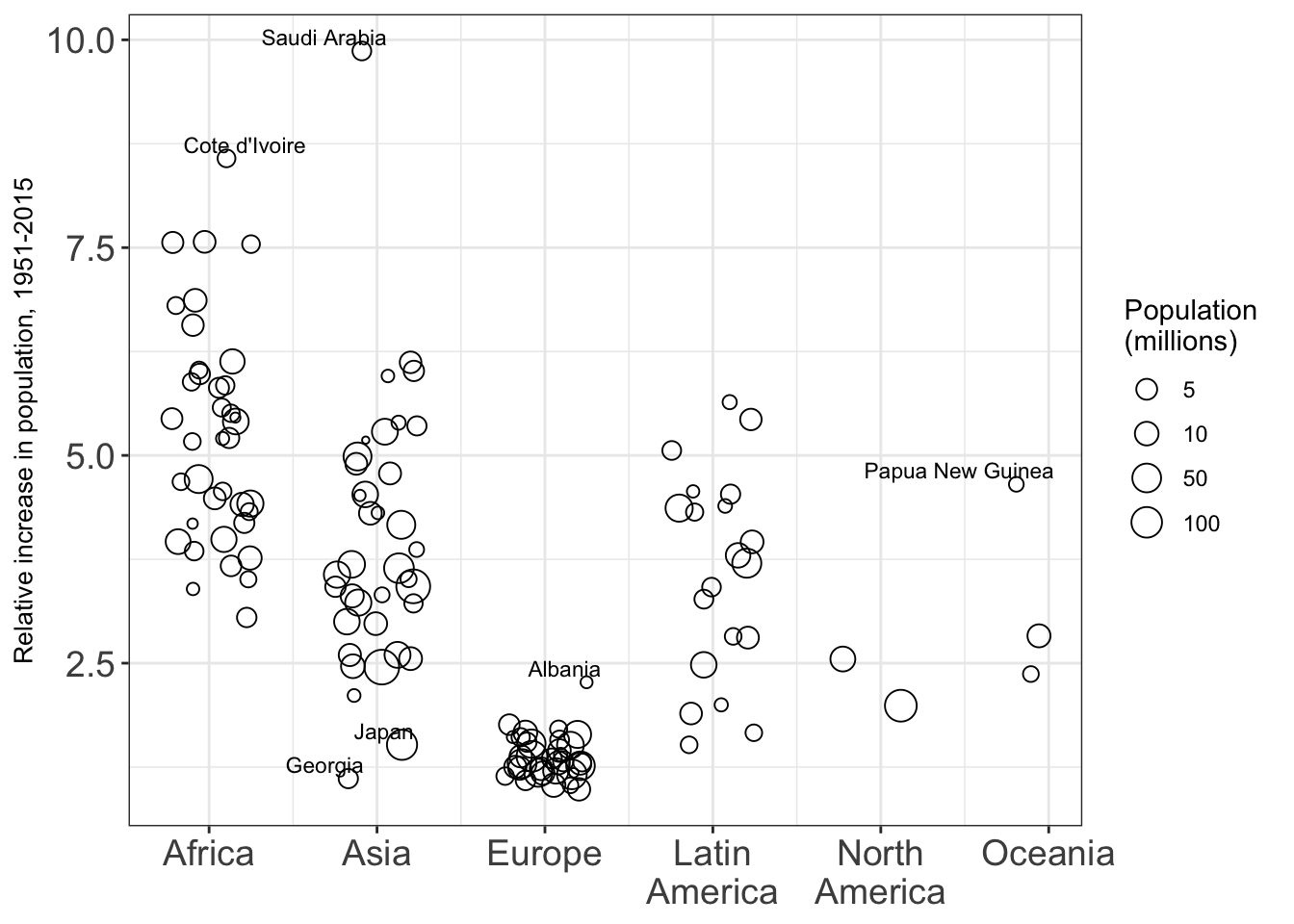Data is from United Nations Population Division: World Population Prospects 2017, specifically Total Population - Both Sexes

#install.packages("ggpubr")
library("magrittr")
library("ggpubr")
library("ggplot2")
library("scales")

# read in data, which I had to transform to numeric format

Country <- as.character(UN.data[,2])
Country = "Cote d'Ivoire"

Pops <- UN.data[, 3:152]/1000 # populations in millions
Years <- 1951:2100

africa=c(15:34,36:44,46:58,60:76) # countries comprising continents
asia=c(79:86,89:93,95:103,105:115,117:134)
europe=c(137:146,148:160,162:177,179:187)
northam=c(241:245)
latinam=c(190:215,217:224,226:239)
oceania=c(248:249,251:255,257:263,265:273)

Continent <- rep(0, nrow(UN.data)) # numeric continent as x-coordinate
Continent[africa] = 1
Continent[asia] = 2
Continent[europe] = 3
Continent[latinam] = 4
Continent[northam] = 5
Continent[oceania] = 6

ContinentNames = c("Africa", "Asia", "Europe", "Latin\nAmerica", "North\nAmerica", "Oceania")

JitterX = Continent - 0.5*(-0.5 + runif(length(Continent))) # jitter continent

Classified = Continent > 0  #exclude non-classified countries
Bigger = Pops[,1] > 1    #retain countries over 1 million in 1950
Keepers = Bigger * Classified > 0 # countries to use

Increase <- Pops[,66] / Pops[,2] # pick out 2015 and 1951

LabelTargets <- c("Cote d'Ivoire", "Saudi Arabia", "Japan", "Georgia", "Albania", "Papua New Guinea")
LabelIndices <- sapply(LabelTargets, function(s){which(Country==s)}) # numbers for labelled countries

GetsLabel <- rep(0, length(Country))
GetsLabel[LabelIndices] = 1

PopRed <- cbind(Pops[Keepers,],
as.factor(Continent[Keepers]),
JitterX[Keepers],
Increase[Keepers],
Country[Keepers],
GetsLabel[Keepers])
names(PopRed) <- c(names(Pops), "ContinentFactor", "Continent", "Increase", "Country", "GetsLabel")

BT <- 14
BiggerTicks <- theme(axis.text.x = element_text(size=BT), axis.title.x = element_text(size=BT),
axis.text.y = element_text(size=BT), axis.title.y = element_text(size=BT))

p <- ggplot(PopRed, aes(x=Continent, y=Increase, label=Country)) # initial plot object, p
p <- p + geom_point(aes(size = X1951), shape=1) # scatter type with circles

p <- p + scale_size_continuous(trans="log10", breaks=c(5,10,50,100)) # defines scatter circle size logarithmicly according to country's population

p <- p + geom_text(aes(label=Country), size=3, hjust=c(0.35,0.8,0.8,0.8,0.8,0.8), vjust=-0.4, data=subset(PopRed, GetsLabel>0)) # defines text labels and positions for the siz countries in LabelTargets list

p <- p + labs(y="Relative increase in population, 1951-2015") # defines y-axis label

p <- p + scale_x_continuous(breaks=1:6, labels=ContinentNames) # x-axis labels

p <- p + guides(size=guide_legend(title="Population \n(millions)")) # labels legend
p <- p + theme_bw() # a complete theme, controls non-data display
p <- p + BiggerTicks # user defined, above, controls axis-text

p <- p + theme(axis.title.x=element_blank())
p <- p + theme(axis.title.y=element_text(size=10))#, face="bold", colour="#990000"))
p # displays the plotFigure 2.8 Relative increase in population between 1951 and 2015 for countries with at least one million people in 1951.